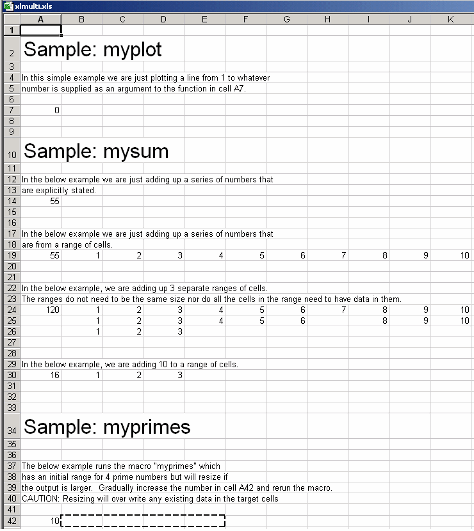Create Macros from MATLAB Functions with Multiple Arguments

This example shows you how to work with, and create macros from, functions that have variable-length inputs and outputs.

Prerequisites

• Verify that you have met all of the MATLAB® Compiler™ Excel® target requirements. For details, see MATLAB Compiler for Microsoft Excel Add-In Prerequisites.

• Verify that you have Microsoft® Excel installed.

• Register mwcomutil.dll on the target machine.

In a system command window, navigate to matlabroot\bin\win64(matlabroot represents the location of MATLAB or MATLAB Runtime on your system), and run the following command:

mwregsvr mwcomutil.dll

• End users must have an installation of MATLAB Runtime to run the add-in. For details, see Install and Configure MATLAB Runtime (MATLAB Compiler SDK).

For testing purposes, you can use an installation of MATLAB instead of MATLAB Runtime.

Procedure

1. Copy the xlmulti example folder that ships with MATLAB to your work folder:

copyfile(fullfile(matlabroot,'toolbox','matlabxl','examples','xlmulti'),'xlmulti')

At the MATLAB command prompt, navigate to the new xlmulti folder in your work folder.

2. Examine the myplot, myprimes, and mysum functions.

myplot takes a single integer input and plots a line from 1 to that number.

function myplot(x)
plot(1:x)

myprimes takes a single integer input n and returns all the prime numbers less than or equal to n.

function p = primes(n)
if length(n)~=1, error('N must be a scalar'); end
if n < 2, p = zeros(1,0); return, end
p = 1:2:n;
q = length(p);
p(1) = 2;
for k = 3:2:sqrt(n)
if p((k+1)/2)
p(((k*k+1)/2):k:q) = 0;
end
end
p = p(p>0);

mysum takes an input of varargin of type integer, adds all the numbers, and returns the result.

function y = mysum(varargin)
y = sum([varargin{:}])

The Microsoft Excel file xlmulti.xls demonstrates these functions in several ways.

3. Build the Excel add-in with the Library Compiler app or compiler.build.excelAddIn using the following information:

FieldValue
Library Namexlmulti
Class Namexlmulticlass
Files to compilemyplot.m
myprimes.m
mysum.m

For example, if you are using compiler.build.excelAddIn, type:

'ClassName','xlmulticlass', ...
'GenerateVisualBasicFile','on');

For more details, see the instructions in Create Excel Add-In from MATLAB.

4. Start Microsoft Excel on your system.

5. Open the file xlmulti.xls.

The example appears as shown:Note

If an Excel prompt says that this file contains macros, click Enable Macros to run this example.

6. To call the function myplot with a value of 4, make A7 (=myplot(4)) the active cell. Press F2 and then Enter.This procedure plots a line from 1 through 4 in a MATLAB Figure window. This graphic can be manipulated similarly to the way one would manipulate a figure in MATLAB. Some functionality, such as the ability to change line style or color, is not available.

The calling cell contains 0 because the function does not return a value.

7. The next illustration calls the function mysum in four different ways:

• The first (cell A14) takes the values 1 through 10, adds them, and returns the result of 55 (=mysum(1,2,3,4,5,6,7,8,9,10)).

• The second (cell A19) takes a range object that is a range of cells with the values 1 through 10, adds them, and returns the result of 55 (=mysum(B19:K19)).

• The third (cell A24) takes several range objects, adds them, and returns the result of 120 (=mysum(B24:K24,B25:L25,B26:D26)). This illustration demonstrates that the ranges do not need to be the same size and that all the cells do not need a value.

• The fourth (cell A30) takes a combination of a range object and explicitly stated values, adds them, and returns the result of 16 (=mysum(10,B30:D30)).This illustration runs when the Excel file is opened. To reactivate the illustration, activate the appropriate cell, then press F2 followed by Enter.

8. In the next illustration, the macro myprimes calls the function myprimes.m with an initial value of 10 in cell A42. The function returns all the prime numbers less than 10 to cells B42 through E42.To execute the macro, from the main Excel window (not the Visual Basic® Editor), open the Macro dialog box by pressing the Alt and F8 keys simultaneously, or by selecting Tools > Macro > Macros.

9. Select myprimes from the list and click .This function automatically resizes if the returned output is larger than the output range specified. Change the value in cell A42 to a number larger than 10. Then rerun the macro. The output returns all prime numbers less than the number you entered in cell A42.Inspect the Microsoft Visual Basic Code (Optional)

1. From the Developer tab, click , or press ALT+F11 to open the Visual Basic Editor. In older versions of Excel, it may be located under Tools > Macro > Visual Basic Editor .

2. In the Project - VBAProject window, double-click to expand VBAProject (xlmulti.xls).

3. Expand the Modules folder and double-click the Module1 module. This opens the VB Code window with the code for this project.